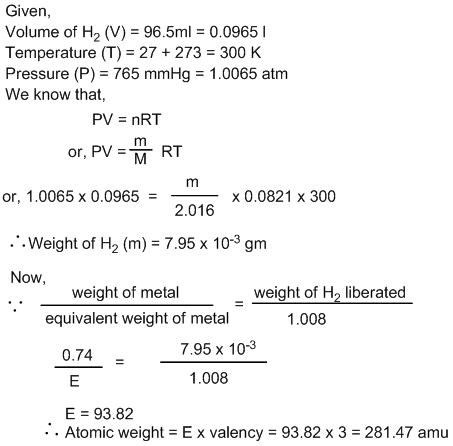• Post Author:

#### Electrochemistry

The branch of physical chemistry which study about the relation between electricity and chemical process involved is called electrochemistry.

#### Electrolytes

Electrolytes are the aqueous solution of chemical substance like acid, base and salt which conduct electricity in aqueous medium.

For examples:- Aqueous solution of H2SO4, NaOH, NaCl, etc.

Electrolytes are ionized into charged particles (ie. Cation and anion) when electricity is passed through them.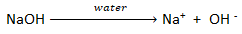Depending upon the strength of electrolytes, they can be classified into two types.

1. Strong electrolytes
2. Weak electrolytes

Strong electrolytes:

The electrolytes which are completely ionized in aqueous medium are called strong electrolytes. It can conduct electricity easily.

Examples- Solution of H2SO4, HNO3, HCl, NaOH, NaCl,etc.

Weak electrolytes:

The electrolytes which are partially ionized in aqueous medium are called weak electrolytes. It conducts electricity partially.

Examples- Solution of CH3COOH, NH4OH, H2CO3, etc.

Non – Electrolytes:

Non – electrolytes are the aqueous solution of chemical substance which do not conduct electricity in aqueous medium.

Examples – Solution of glucose, sugar, urea etc.

#### Electrolysis

The process of chemical decomposition of an electrolyte in solution by using electric current is called electrolysis.

This process is carried out in a vessel called electrolytic cell or voltameter. The two metallic rods are connected to two terminals of battery in electrolytic solution with the help of electric wire. These metallic rods are called electrodes. The electrode connected to positive terminal of battery is called anode and the electrode connected to negative terminal of battery is called cathode. In cathode reduction takes place whereas in anode oxidation takes place.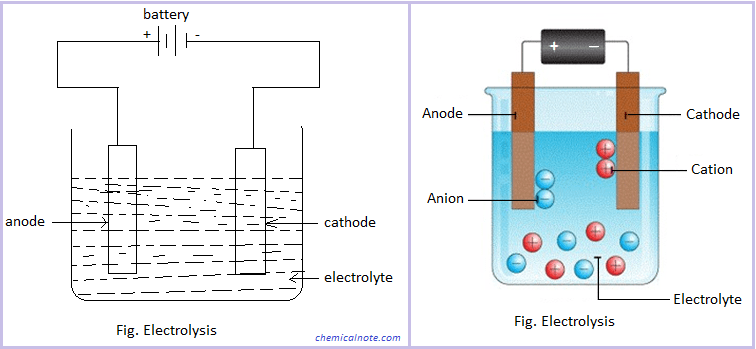If the electrolyte is NaCl solution, then

At cathode : ReductionAt anode : Oxidation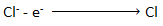Applications of Electrolysis

• Electrolysis can be used to extract the pure metals in electroplating, electro refining, etc.
• It can be used to manufacture oxygen and hydrogen gas from water.

#### Faraday’s laws of electrolysis

##### Faraday’s first law of electrolysis :

This law states that, “The mass of the substance deposited (discharged) at the electrode during electrolysis is directly proportional to the quantity of electricity passed through the solution.”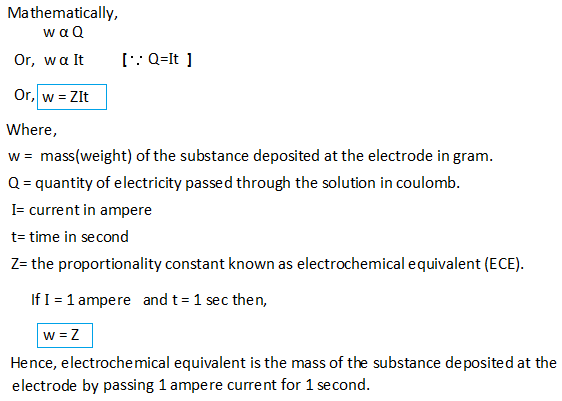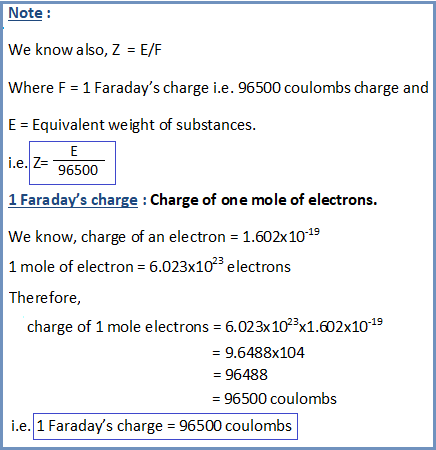Faraday’s Second law of electrolysis

This law states that “the mass(weight) of different substances deposited or liberated at electrode by the same amount of electricity is directly proportional to the equivalent weight of substances”.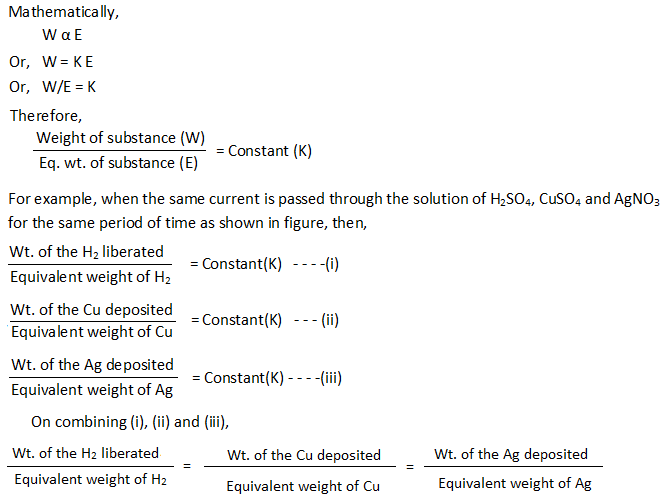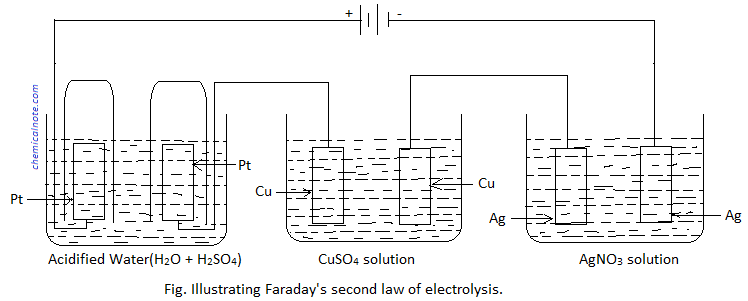It is found that when 1.008 gram of H2 is evolved from acidified water, then the masses of copper and silver deposited are 31.75 gram and 108 gram respectively, which are the equivalent weight of copper and silver respectively. Hence, this verifies Faraday’s second law of electrolysis.

#### Numerical Problems

1. How many Faraday of electricity are there in 6.0 X 1012 electrons?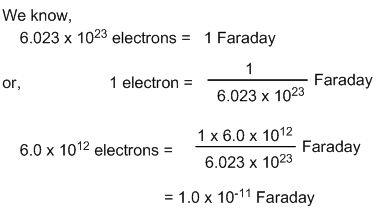2. How many coulombs of electricity is required for the following changes?

a. 1 mole of Al3+ to Al

b. One mole of H2O to O2

c. One mole of MnO4 to Mn2+

d. 1 mole of FeO to Fe2O3

e. 1 mole of Cr2O7– – to Cr3+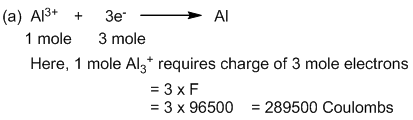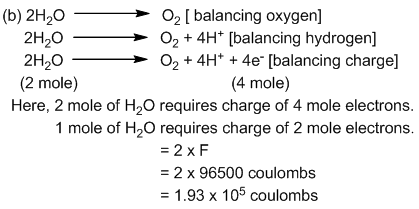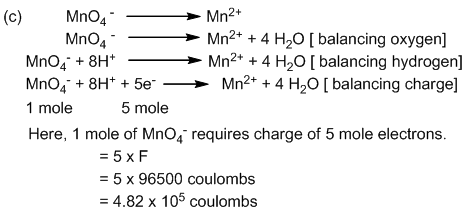Ans. (d) 96500 (e) 5.79X105

3. Find the charge in coulomb in 1 g ion of N3-.

A gram-ion means the number of ions that are present in 1 mole of the substance. NN has only 1 gram-ion since it forms only one ion i.e., N3− at a time.
Therefore, 1g-ion of N3− means 1 mole of N3− .

There are 3 electrons in this ion, hence, 3 moles of electrons are present in 1g-ion or 1 mole of N3−.
Charge present on 1 mole of electrons = 96500 C
Charge present on 3 moles of electrons = 96500×3 C
Therefore, charge in 1g ion of N3- is 2.895×105C

4. What current strength in amperes will be required to liberate 10g iodine from potassium iodide solution in one hour? (at.wt. of iodine = 127).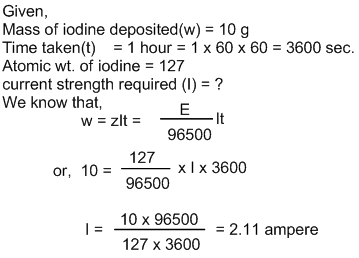5. A current of 2.5 ampere passes through the solution of zinc sulphate for 30 minutes and deposits 1.52 g of zinc metal at cathode. What is the equivalent weight of zinc metal?

[Ans = 32.59]

6) 0.2964 g of copper was deposited by the passage of a current of 0.5 amperes for 30 minutes through a solution of CuSO4. Calculate the atomic weight of copper.

[Ans = 63.56]

7) How long a current of 3 amperes has to be passed through a solution of AgNO3 to coat a metal surface of 80cm2 with 0.005mm thick layer.(Density of silver = 10.5g/cc and at.wt. of Ag = 107.7)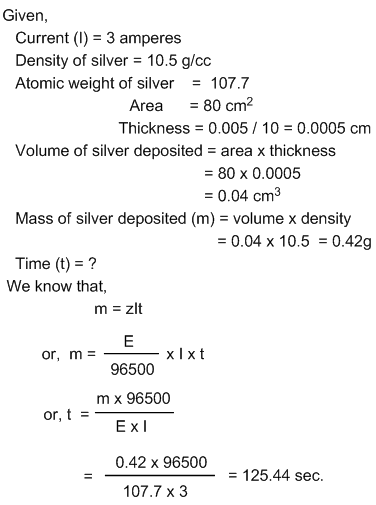8) A metallic spoon is coated with silver by passing a current of 5 amperes through AgNO3 solution for 5 hours. What is the thickness of silver plating if the area of spoon is 12 cm2. [Given, density of silver is 10.5 g/cc, atomic weight of Ag is 107.92]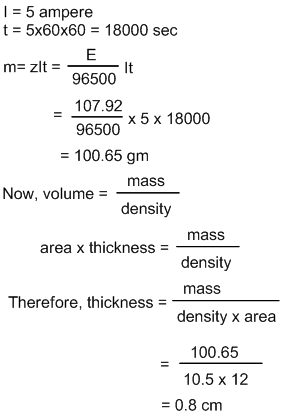9) Silver is deposited on a metallic vessel of surface area 800 cm2 by passing a current 0.2 amperes for 3 hours. Calculate the thickness of silver deposited. Given the density of silver as 10.47g/cc. [atomic mass of Ag = 107.92]

{Ans. 2.88 x 10-4 cm}

10) Iron slab having dimension 50cm x 30cm x 20cm was electroplated all around the surface with a uniform thickness of copper by passing 5 ampere of current for 2 hours in CuSO4 solution. Find the thickness of Cu deposited. Given the specific gravity of Cu = 8.9 and its atomic mass = 63.5

[Hint: Here, total surface area = 2[50×30+50×20+30×20]

Specific gravity = density]

11) Find out the number of coulombs needed to plate a rectangular area of 25cm x 4cm with thickness of 10-2 cm using CuSO4 as electrolyte. Density of Cu is 8.94 g/ml.

[Ans. 27171.96 coulombs]

12) How many molecules of chlorine should be deposited from molten sodium chloride in one minute by a current of 300 milli amperes ?

[ans. 5.616×1019molecules]

13. If 5A current is supplied through zinc sulphate solution till 12 minutes deposits 1.2g of zinc, find the current efficiency.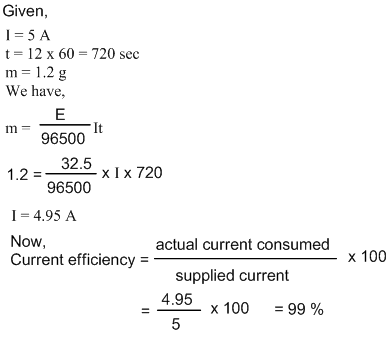14) A solution of CuSO4 is electrolyzed by passing a current of 360mA for 35.3 minutes. Calculate the mass of metal deposited if current efficiency was 60% (at. Mass of Cu=63.5).

[Ans. 0.15g]

15) If the cost of electricity to deposit 5 g Mg from its salts solution is Rs. 25, find the cost of the electricity to deposit equal quantity of Zn from its salt solution.

16) Calculate the volume of Cl2 at NTP produced during electrolysis of MgCl2 which produces 6.50g Mg. (At.wt. of Mg=24.3)

17) An electric current is passed through three cell in series containing solution of copper sulphate, silver nitrate and potassium iodide respectively. What weight of silver and iodine will be liberated when 1.25g of copper is being deposited.

[Ans. 4.269g, 5g]

18) An electric current is passed between platinum electrode through a solution of silver nitrate, copper sulphate and dilute sulphuric acid solution in series. If one gram of hydrogen is evolved by this current in the third cell, calculate (i) the weight of silver and copper deposited in the first and second cell (ii) the volume of hydrogen measured at STP which is liberated from the third cell. [ eq.wt. of H = 1, Cu = 31.5 and Ag = 108]

19) Equal amount of current was passed through an aqueous solution of trivalent metallic salt and dil. H2SO4. The volume of H2 liberated was 96.5ml at 270C and 765mmHg and weight of metal deposited was 0.74gm. Calculate the atomic weight of metal.